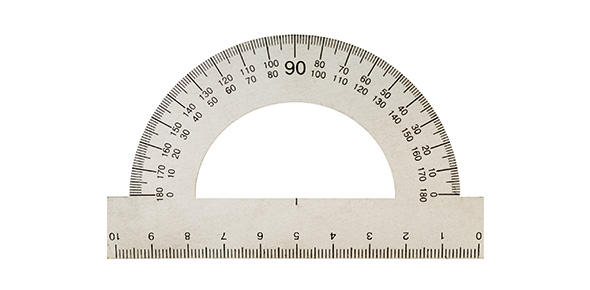# Vsol 6.9 Customary Units

10 Questions | Total Attempts: 77SettingsCourse 1 assessment on converting units in the Customary System of measurement.

• 1.
What is 15 yd minus 4 ft?
• A.

19 ft

• B.

41 ft

• C.

40 ft

• D.

11 ft

• 2.
A wall is 12 ft long.  How long is the wall in inches?
• A.

4 in

• B.

36 in

• C.

48 in

• D.

144 in

• 3.
Which is equivalent to 5 miles?
• A.

3,168 in

• B.

31,680 in

• C.

316,800 in

• D.

3,168,000 in

• 4.
An elephant can weigh as much as 15,000 pounds.  How many tons could an elephant weigh?
• A.

7 1/2 T

• B.

15 1/2 T

• C.

70 1/2 T

• D.

150 1/2 T

• 5.
Jamal's baby brother weighs 8 1/2 pounds.  How many ounces does his brother weigh?
• A.

128 oz

• B.

136 oz

• C.

132 oz

• D.

130 oz

• 6.
Which is equivalent to 2 1/4 tons?
• A.

720 oz

• B.

4,500 oz

• C.

4,50 lbs

• D.

7,200 oz

• 7.
Jake bought 5 pounds of peanuts for \$17.50.  How much do 8 ounces of peanuts cost?
• A.

\$1.50

• B.

\$1.75

• C.

\$8.00

• D.

\$12.00

• 8.
A cereal snack mix contains 4 1/4 cups of bran cereal, 2 2/3 cups of wheat cereal and 1 1/4 cups of corn cereal.  What is the total amount of cereal in the snack mix?
• A.

7 1/12 cups

• B.

8 1/6 cups

• C.

8 cups

• D.

7 1/6 cups

• 9.
Marissa bought 1 3/8 pounds of nuts.  She ate 1/4 pound of the nuts and her brother ate 1/8 pound.  How many pounds of nuts are left?
• A.

1 lb

• B.

3/4 lb

• C.

1 1/4 lb

• D.

3/8 lb

• 10.
A water jug contains 14 1/2 quarts of water.  How many cups of water are in the jug?
• A.

29 cups

• B.

56 cups

• C.

58 cups

• D.

464 cups

Related TopicsBack to top